# class ROOT::Math::RichardsonDerivator

```
User class for calculating the derivatives of a function. It can calculate first (method Derivative1),
second (method Derivative2) and third (method Derivative3) of a function.

It uses the Richardson extrapolation method for function derivation in a given interval.
The method use 2 derivative estimates (one computed with step h and one computed with step h/2)
to compute a third, more accurate estimation. It is equivalent to the
<a href = http://en.wikipedia.org/wiki/Five-point_stencil>5-point method</a>,
which can be obtained with a Taylor expansion.
A step size should be given, depending on x and f(x).
An optimal step size value minimizes the truncation error of the expansion and the rounding
error in evaluating x+h and f(x+h). A too small h will yield a too large rounding error while a too large
h will give a large truncation error in the derivative approximation.
A good discussion can be found in discussed in
<a href=http://www.nrbook.com/a/bookcpdf/c5-7.pdf>Chapter 5.7</a>  of Numerical Recipes in C.
By default a value of 0.001 is uses, acceptable in many cases.

This class is implemented using code previosuly in  TF1::Derivate{,2,3}(). Now TF1 uses this class.

@ingroup Deriv

```

## Function Members (Methods)

public:
 ~RichardsonDerivator() double Derivative1(double x) double Derivative1(const ROOT::Math::IGenFunction& f, double x, double h) double Derivative2(double x) double Derivative2(const ROOT::Math::IGenFunction& f, double x, double h) double Derivative3(double x) double Derivative3(const ROOT::Math::IGenFunction& f, double x, double h) double Error() const double operator()(double x) ROOT::Math::RichardsonDerivator& operator=(const ROOT::Math::RichardsonDerivator& rhs) ROOT::Math::RichardsonDerivator RichardsonDerivator(double h = 0.001) ROOT::Math::RichardsonDerivator RichardsonDerivator(const ROOT::Math::RichardsonDerivator& rhs) ROOT::Math::RichardsonDerivator RichardsonDerivator(const ROOT::Math::IGenFunction& f, double h = 0.001, bool copyFunc = false) void SetFunction(const ROOT::Math::IGenFunction& f) void SetStepSize(double h)

## Data Members

protected:
 const ROOT::Math::IGenFunction* fFunction pointer to function bool fFunctionCopied flag to control if function is copied in the class double fLastError error estimate of last derivative calculation double fStepSize step size used for derivative calculation

## Class Charts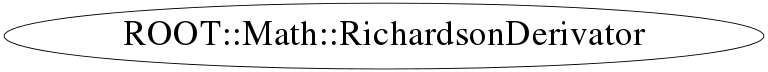## Function documentation

``` Destructor: Removes function if needed.
```
RichardsonDerivator(double h = 0.001)
``` Default Constructor.
Give optionally the step size for derivation. By default is 0.001, which is fine for x ~ 1
Increase if x is in averga larger or decrease if x is smaller

```
RichardsonDerivator(const ROOT::Math::IGenFunction& f, double h = 0.001, bool copyFunc = false)
``` Construct from function and step size

```
RichardsonDerivator(const ROOT::Math::RichardsonDerivator& rhs)
```      Copy constructor

```
RichardsonDerivator & operator=(const ROOT::Math::RichardsonDerivator& rhs)
```      Assignment operator

```
double Error() const
``` Returns the estimate of the absolute Error of the last derivative calculation.
```
`{ return fLastError; }`
double Derivative1(double x)
```      Returns the first derivative of the function at point x,
computed by Richardson's extrapolation method (use 2 derivative estimates
to compute a third, more accurate estimation)
first, derivatives with steps h and h/2 are computed by central difference formulas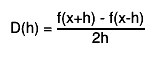$D(h) = #frac{f(x+h) - f(x-h)}{2h}$
the final estimate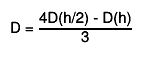$D = #frac{4D(h/2) - D(h)}{3}$
"Numerical Methods for Scientists and Engineers", H.M.Antia, 2nd edition"

the argument eps may be specified to control the step size (precision).
the step size is taken as eps*(xmax-xmin).
the default value (0.001) should be good enough for the vast majority
of functions. Give a smaller value if your function has many changes
of the second derivative in the function range.

Getting the error via TF1::DerivativeError:
(total error = roundoff error + interpolation error)
the estimate of the roundoff error is taken as follows: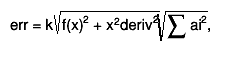$err = k#sqrt{f(x)^{2} + x^{2}deriv^{2}}#sqrt{#sum ai^{2}},$
where k is the double precision, ai are coefficients used in
central difference formulas
interpolation error is decreased by making the step size h smaller.

```
double operator()(double x)
`{ return Derivative1(x); }`
double Derivative1(const ROOT::Math::IGenFunction& f, double x, double h)
```      First Derivative calculation passing function and step-size

```
double Derivative2(double x)
```      Returns the second derivative of the function at point x,
computed by Richardson's extrapolation method (use 2 derivative estimates
to compute a third, more accurate estimation)
first, derivatives with steps h and h/2 are computed by central difference formulas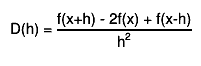$D(h) = #frac{f(x+h) - 2f(x) + f(x-h)}{h^{2}}$
the final estimate$D = #frac{4D(h/2) - D(h)}{3}$
"Numerical Methods for Scientists and Engineers", H.M.Antia, 2nd edition"

the argument eps may be specified to control the step size (precision).
the step size is taken as eps*(xmax-xmin).
the default value (0.001) should be good enough for the vast majority
of functions. Give a smaller value if your function has many changes
of the second derivative in the function range.

Getting the error via TF1::DerivativeError:
(total error = roundoff error + interpolation error)
the estimate of the roundoff error is taken as follows:$err = k#sqrt{f(x)^{2} + x^{2}deriv^{2}}#sqrt{#sum ai^{2}},$
where k is the double precision, ai are coefficients used in
central difference formulas
interpolation error is decreased by making the step size h smaller.

```
double Derivative2(const ROOT::Math::IGenFunction& f, double x, double h)
```      Second Derivative calculation passing function and step-size

```
double Derivative3(double x)
```      Returns the third derivative of the function at point x,
computed by Richardson's extrapolation method (use 2 derivative estimates
to compute a third, more accurate estimation)
first, derivatives with steps h and h/2 are computed by central difference formulas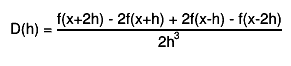$D(h) = #frac{f(x+2h) - 2f(x+h) + 2f(x-h) - f(x-2h)}{2h^{3}}$
the final estimate$D = #frac{4D(h/2) - D(h)}{3}$
"Numerical Methods for Scientists and Engineers", H.M.Antia, 2nd edition"

the argument eps may be specified to control the step size (precision).
the step size is taken as eps*(xmax-xmin).
the default value (0.001) should be good enough for the vast majority
of functions. Give a smaller value if your function has many changes
of the second derivative in the function range.

Getting the error via TF1::DerivativeError:
(total error = roundoff error + interpolation error)
the estimate of the roundoff error is taken as follows:$err = k#sqrt{f(x)^{2} + x^{2}deriv^{2}}#sqrt{#sum ai^{2}},$
where k is the double precision, ai are coefficients used in
central difference formulas
interpolation error is decreased by making the step size h smaller.

```
double Derivative3(const ROOT::Math::IGenFunction& f, double x, double h)
```      Third Derivative calculation passing function and step-size

```
void SetFunction(const ROOT::Math::IGenFunction& f)
``` Set function for derivative calculation (copy the function if option has been enabled in the constructor)

\@param f Function to be differentiated

```
void SetStepSize(double h)
``` Set step size for derivative calculation

\@param h step size for calculation

```
`{ fStepSize = h; }`